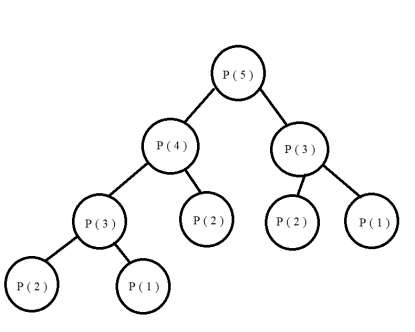# Count Derangements (Permutation such that no element appears in its original position) in C++

Derangement is permutation of N numbers such that no number appears at original position. For example one possible derangement of { 1,2,3 } is { 2,1,3 }. No element in this is at its original position. The goal here is to count the possible derangements for N numbers.

We will do this using a recursive solution. For following no. of elements −

• N=0, no derangement, return 1
• N=1, only one number, return 0
• N=2, only one swap of position possible, { 1,2 } → { 2,1 }, return 1
• N=3, 2 possible permutations eg, { 1,2,3 } → { 2,3,1 }, { 3,1,2 } count 2
• N=4, 9 possible permutations
• .......................
• N, (N-1)*( permutation(N-1) + permutation(N-2) )

For all elements in an array,

• Element at index 0 has n-1 positions that it can take,

• If any element at index i is placed at index 0 then, arr[i] and arr are swapped. Now n-2 elements are there for calculation.

• If element at index i is not placed at index 0 then for n-1 elements there are n-2 choices.

Diagram## Input

Arr[] = { 1, 2 }

## Output

No. of derangements : 1

Explanation − Positions of 1 and 2 are index 0 and 1. Only position they can get is swapping original positions. { 2,1 }

## Input

Arr[] = { 1, 2, 3 }

## Output

No. of derangements : 2

Explanation − Positions of 1,2 and 3 are index 0,1,2.

1 can be placed at index 1 and 2, 2 can be placed at index 0 and 3 and 3 can be placed at index 0 and 1.

{ 2,3,1 } and { 3,1,2 } are 2 derangements.

## Approach used in the below program is as follows

• Integer Num stores the count of numbers present.

• Recursive function derangements(int N) takes the count of numbers as input and returns the no. of derangements.

• The return statements for N=0,1,2 are handling the base cases for which permutations are already calculated as 1,0 and 1.

• When N>2 then recursive calls to derangements() is done using the formula,

(N-1)* ( derangements ( N-1) + derangements ( N-2) ).

When backtracking starts, the count is computed and returned.

## Example

Live Demo

#include <bits/stdc++.h>
using namespace std;
int derangements(int N){
if (N == 0)
return 1;
if (N == 1)
return 0;
if (N == 2)
return 1;
return (N - 1) * (derangements(N - 1) + derangements(N - 2));
}
int main(){
int Numbers=5;
cout<<"Number of Derangements :"<<derangements(Numbers);
}

## Output

Number of Derangements :44